Pascal Implementation by Steven Pemberton and Martin Daniels

# Chapter 10: The P-code Machine

The P-code machine is similar to a conventional computer in that it consists of a processor and a memory. A major difference is that many operations performed by the processor involve the stack which is part of memory. For example a procedure call entails manipulating various factors such as parameters and return addresses and these are held on the stack. The machine instructions, called P-code, are stored in the memory and accessed in the normal manner.

The processor has a defined instruction set (detailed later) as well as five registers which have distinct functions for controlling the instructions and the stack areas within the memory.

The registers are:

• `PC` the program counter;
• `SP` the stack pointer
• `MP` the mark stack pointer;
• `NP` the new pointer;
• `EP` the extreme stack pointer.

The program counter, `PC`, is a pointer to the current instruction being executed. The other registers are used to control the remaining area of memory - the data store.

The data store is divided into three areas as shown in Fig. 10.1.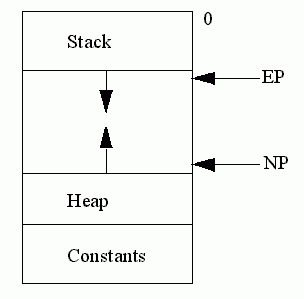Fig. 10.1

The constants area is generated by the assembler and accessed by the code (details may be found in the next chapter)

The heap grows towards the low-numbered locations of store and free heap space is pointed at by the `NP` pointer. Data is put onto the heap during a call to the new procedure and removed by using mark and release. The stack area has a further internal structure and is used to hold stack frames. A stack frame is generated and placed on the stack each time a procedure or function is called (in the following section, except where explicitly stated, references to procedures also include functions.) The first stack frame is the exception in that this belongs to the program block. The stack frames on the stack are shown in Fig. 10.2.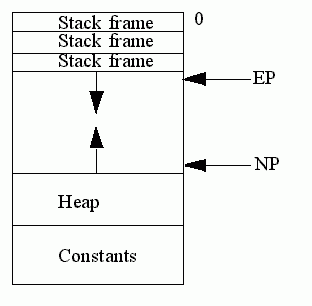Fig. 10.2

### The Structure of Stack Frames

The stack frame format with its associated registers is shown in Fig 10.3.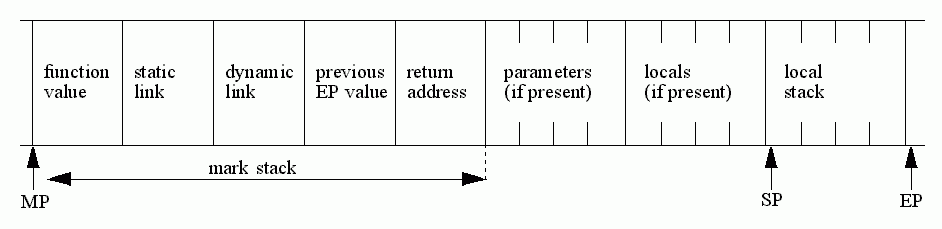Fig. 10.3

The mark stack pointer, `MP`, points to the base of the topmost stack frame and is used to regain the stack space when the execution of a procedure is complete and as a base to access local variables. The extreme stack pointer, `EP`, points to the top of the current stack frame. It protects the stack frame from `NP`, so that `SP` does not have to be checked each time it is incremented, and it also marks the point where a new stack frame can be placed should a procedure be called from the current one. The maximum possible size of each stack frame is known at compile time and this is reflected by the value of `EP`.

The local stack is used for holding temporary values during the execution of a procedure. For example the `SBI` instruction takes the two values from the top of this stack, subtracts them and returns this value back to the stack. The stack pointer will be adjusted to reflect the fact that there is now one value less on the stack.

The locals area is used for variables that have been declared within a procedure and therefore whose scope is local. For example consider the following procedure and the corresponding P-code:

```program locals(input, output);
var i: integer; (*The variable i is local to the procedure addlocal*)
begin
i:=i+1
begin
end.```
```L 3        Start of addlocal
ENT 1 L 4
ENT 2 L 5
LODI 0 5  The variable i retrieved from the first location above the mark stack i.e. 5
LDCI 1
STRI 0 5  New value of i is stored back in 5
L 4= 6
L 5= 7
L 6
I 10
ENT 1 L 7
ENT 2 L 8
MST 0
CUP 0 L 3 Procedure addlocal is called
RETP
L 7= 9
L 8= 5
Q
I0
MST 0
CUP 0 L 6 Main program block is entered
STP
Q```

The parameters area is used for transferring procedure parameters from the calling block; it is an address if the parameter is a 'call-by-reference' otherwise it is the value itself. The following example illustrates how parameters are passed:

```
program params(input, output);
var j: integer;

begin
i:=i+1

begin
j:=1;
end.```
```L 3
ENT 1 L 4
ENT 2 L 5
LODA 0 5
LODA 0 5  The address of i
INDI 0    Load indirect the contents of i
LDCI 1
I 10
STOI
RETP
L 4= 6
L 5= 8
L 6
ENT 1 L 7
ENT 2 L 8
LDCI 1
SROI 9
MST 0
LAO 9     Address of j, to be used as a parameter
CUP 1 L 3 Call to addparam
RETP
L 7= 10
L 8= 6
Q

I0
MST 0
CUP 0 L 6
STP
Q```

The mark stack carries the administrative details for executing and returning from a procedure and for maintaining the necessary links to access variables in outer levels:

• the function value is simply an area used for returning the function value to the calling block and so is only used by functions; in practice this area must be capable of holding the largest possible value that may be returned by a function;
• the static link is used as a pointer to access variables in outer blocks; it points to the base of the stack frame of the procedure that textually encloses it;
• the dynamic link is the previous `MP` value so that the current stack frame may be removed when the execution of the called procedure is complete;
• the previous EP value is similarly used for resetting the `EP` register when this procedure returns;
• the return address enables program control to be returned to the calling procedure.

The following example program contains a nested recursive procedure and the schematic diagram shows the static and dynamic links on the stack at the time the program halts. The static link of a procedure will always point to the stack frame of the textually enclosing procedure (q will always point to p, p will always point to the program); the dynamic link will always point to the stack frame of the calling procedure (in this example, q will point sometimes to p and sometimes to q):

```program main(input,output);
var j: integer;

procedure p;
var i: integer;

procedure q;
begin
if i > 0 then begin
i:=i-1; j := j+1;
q (*procedure q is called recursively here*)
end
else write(input, 0) (*execution error to halt program*)
end;

begin
i:=2;
q
end;

begin
j := 0;
p
end.```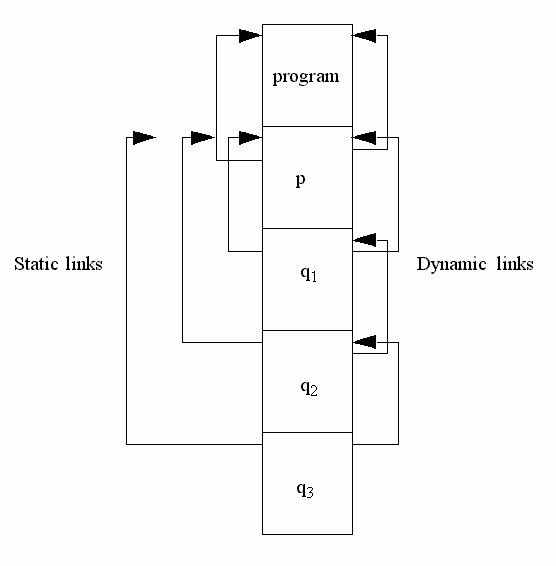Fig. 10.4

If p were called recursively before calling q, the static links of q would then point to the most recent stack frame of p.

### Instructions for calling procedures and functions

There are three P-code instructions for building stack frames, `MST` and `CUP` are used before entering the procedure and `ENT` is used for the first two instructions within the procedure. The detailed operation of these instructions is as follows.

A typical calling sequence is

```
MST 0
CUP 0 L 3```

The operand of the `MST` (mark stack) instruction is an indication of the depth of nesting of the given procedure and is defined as "one plus the level of the calling procedure minus the level of the called procedure". This is used for calculating the static link. In the above example, when the program calls p is will use `MST 0`; when p calls q it will also use a `MST 0`; when q calls itself, it will use `MST 1`.

`MST 0` means: the static link is the stack frame that called you; `MST 1` means the static link is the static link of the stack frame that called you; `MST 2` would use the static link of that frame, and so on.

The execution of `MST` creates values for the static and dynamic links and saves the `EP`. As just explained, it assigns the value of the static link to point to the stack frame of the procedure that textually encloses this one. The dynamic link is assigned the current value of the mark stack pointer and the current value of the extreme stack pointer is stored. The stack pointer is incremented so as to point at the parameter area. This is in readiness for subsequent instructions which may load parameters.

The `CUP` (call user procedure) instruction sets the new value of `MP` and the link for the return address and finally causes the jump to the procedure concerned.

At the start of the procedure there are two `ENT` instructions which define the overall size of the stack frame and adjust `SP` and `EP` accordingly.

```
ENT 1 L 7
ENT 2 L 8```

The labels `L 7` and `L 8` point to the values to be used by the `ENT` instructions.

The procedure execution may now proceed. At conclusion of the procedure, the `RET` (return) instruction provides the mechanism for returning to the calling procedure and removing stack frames.

The 'main' program block is handled in the same way as procedures as regards the stack, except that there are four locations reserved above the mark stack for files. These locations have fixed addresses as shown in Fig. 10.5, and are manipulated in the same way as global variables. The files may be regarded as parameters to the program.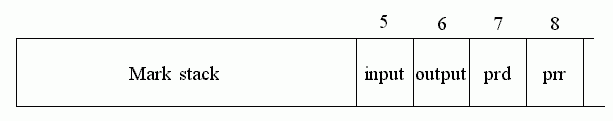Fig. 10.5

Thus the reference in a Pascal program to a file variable for example input^ , will be a direct reference to the contents of location 5.

### The P-Code Instruction Set

The following table describes the complete instruction set showing the parameters and the effect of the execution of each instruction on the stack. Only a brief description of each instruction is given here - a detailed version is given in the chapter on the interpreter.

Instruction Operation on stack Parameters if present Description of instruction
Before After
ABI (i) i   Absolute value of integer
ABR (r) r   Absolute value of real
ADI (i,i) i   Adds two integers on the top of the stack and leaves an integer result
ADR (r,r) r   Adds two reals on the top of the stack and leaves a real result
CHKc No change PQ Checks value is between upper and lower bounds
CHR (i) c   Converts integer to character
CSP Special Q Call standard procedure
CUP Special PQ Call user procedure
DECc (x) x Q Decrement
DIF (s, s) s   Set difference
DVI (i,i) i   Integer division
DVR (r,r) r   Real division
ENT Special PQ Enter block
EOF (a) b   Test on end of file
EQUc (x,x) b Q Compare on equal
FJP (b)     False jump
FLO (i,r) r,r   Float next to the top
FLT (i) r   Float top of the stack
GEQc (x,x) b Q Compare on greater or equal
INCc (x) x Q Increment
INDc (a) x Q Indexed fetch
INN (i,s) b   Test set membership
INT (s,s) s   Set intersection
IOR (b,b) b   Boolean inciusive OR
IXA (a,i) a Q Compute indexed address
LCI   x PQ Load constant indirect - assembler generated
LEQc (x,x) b Q Compare on less than or equal
LESc (x,x) b Q Compare on less than
MOD (i,i) i   Modulo
MOV (a,a)   Q Move
MPI (i,i) i   Integer multiplication
MPR (r,r) r   Real multiplication
MST Special P Mark stack
NEQc (x,x) b Q Compare on not equal
NOT (b) b   Boolean not
ODD (i) b   Test on odd
ORDc (x) i   Convert to integer
RETc Special   Return from block
SBI (i,i) i   Integer subtraction
SBR (r,r) r   Real subtraction
SGS (i) s   Generate singleton set
SQI (i) i   Squareinteger
SQR (r) r   Square real
SROc (x)   Q Store at base level address
STOc (a,x)     Store at base level address
STP

No effect

Stop
STRc (x)   PQ Store at level P
TRC (r) i   Truncate
UJC

No effect

Error in case statement
UJP

No effect

Q Unconditional jump
UNI (s, s) s   Set union
XJP (i)   Q Indexed jump

Key to effect on stack: